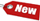Browse Accounting Lessons HereAccounting Terms & DefinitionsAccounting for Merchandising ActivitiesDebits and Credits (Double Entry Accounting)Business Valuation FormulasTime Value of Money & Present/Future ValuesComplex Debt & Equity InstrumentsCommon Stock & Shareholder's EquityAccounting & Finance RatiosValuing Common StockCorporate Income TaxesLower of Cost or Market (LCM) & Inventory ValuationChart of Accounts & BookkeepingBonds Payable & Long Term LiabilitiesCapital AssetsGAAP, Accrual & Cash Accounting, Information Commodity, Internal Controls & MaterialityWhat category of browser are you on this website? Accounting student (homework help) Finance professor (university research) Accounting manager (at work) Other Explore Careers in Accounting and FinanceVisit our section on Careers in Accounting & Finance to explore vast opportunities in this industry.

Chapter 4.5® - Examples of Interest Rate Calculations & Practice Questions #1 - #7

1) Question

A person opens a bank account with a deposit of \$150. At the end of 3 years, there is \$179.34 in the account. What nominal interest rate compounded quarterly was earned on the investment?

 N = 3 I/Y = ? PV = \$150 PMT = \$0 FV = \$179.34 2ND I/Y P/Y = 1 C/Y = 4 CPT & I/Y = 6.14%

2) Question

A deposit of \$1500 earned \$672.45 interest over 7 and ½ years. What nominal interest rate compounded semi-annually was paid on the deposit?

 N = 7.5 I/Y = ? PV = -\$1,500 PMT = \$0 FV = \$2,172.45 2ND I/Y P/Y = 1 C/Y = 2 CPT & I/Y = 5%

3. Question

A principal of \$7100 has maturity value of \$13,966.70 in 10 years. If the interest rate is compounded annually, what is the nominal rate?

 N = 10 I/Y = ? PV = -\$7,100 PMT = \$0 FV = \$13,966.70 2ND I/Y P/Y = 1 C/Y = 1 CPT & I/Y = 6.999% (7%)

4. Question

A present value of \$1301.69 has a future value of \$2569.26 in 7 years and 7 months. What is the nominal rate compounded monthly?
Note: 7 years and 7 months = (7 x 12 months) + 7 months = 91 months

 N = 91 I/Y = ? PV = -\$1,301.69 PMT = \$0 FV = \$2,569.26 2ND I/Y P/Y = 12 C/Y = 12 CPT & I/Y = 9%

5. Question

At what nominal rate compounded quarterly will money double in 7 and ½ years?

 N = 7.5 I/Y = ? PV = -\$100 PMT = \$0 FV = \$200 2ND I/Y P/Y = 1 C/Y = 4 CPT & I/Y = 9.35%

6. Question

At what nominal rate compounded monthly will money double in 6 years?

 N = 6 I/Y = ? PV = -\$100 PMT = \$0 FV = \$200 2ND I/Y P/Y = 1 C/Y = 12 CPT & I/Y = 11.61%

7. Question

At what nominal rate compounded semi-annually will money triple in 9 and ½ years?

 N = 9.5 I/Y = ? PV = -\$100 PMT = \$0 FV = \$300 2ND I/Y P/Y = 1 C/Y = 2 CPT & I/Y = 11.91%